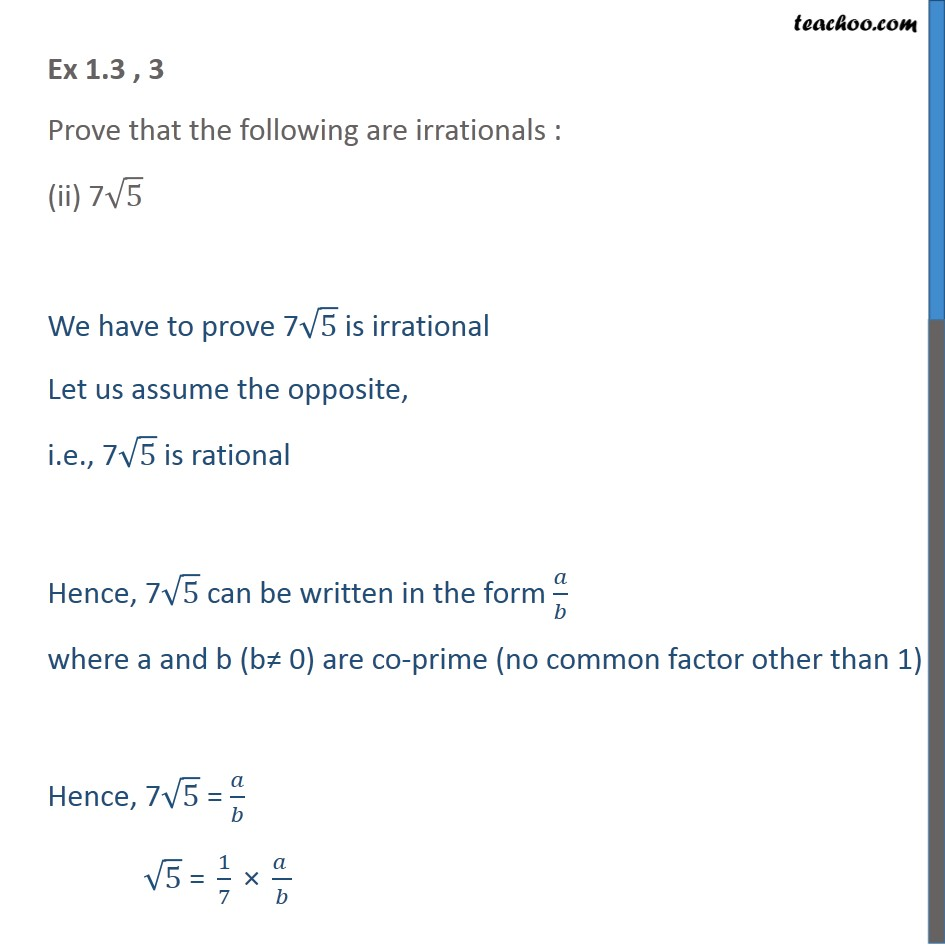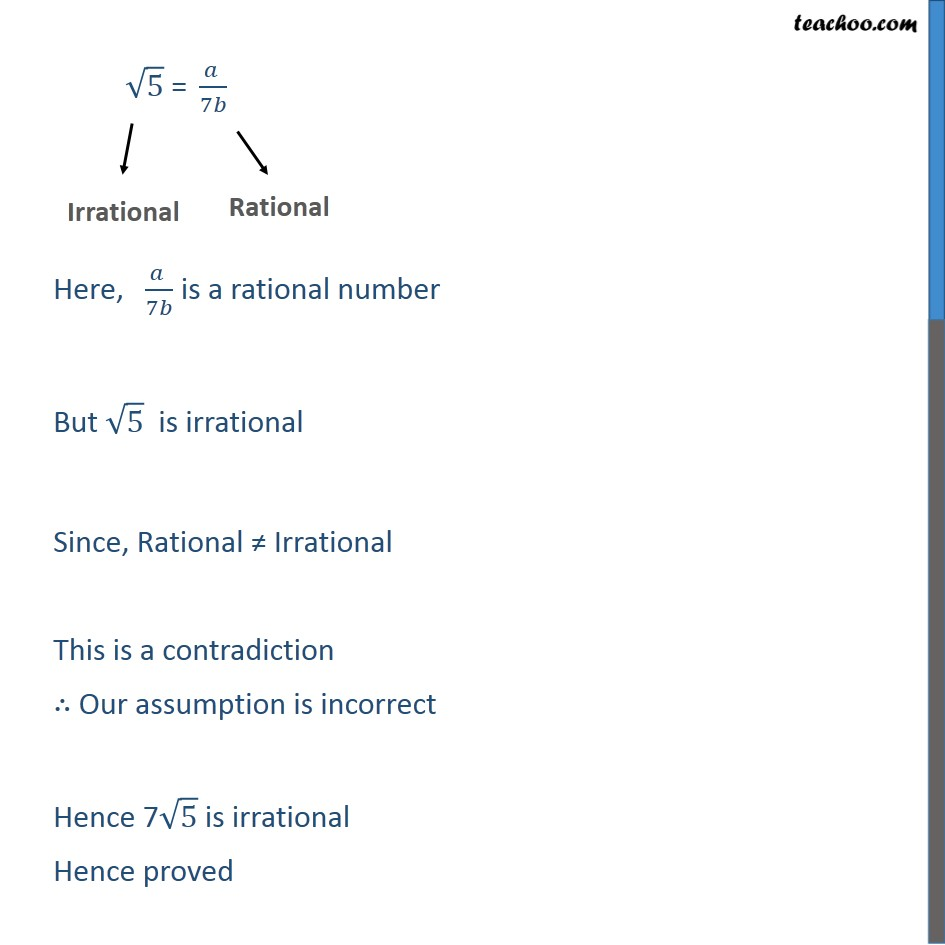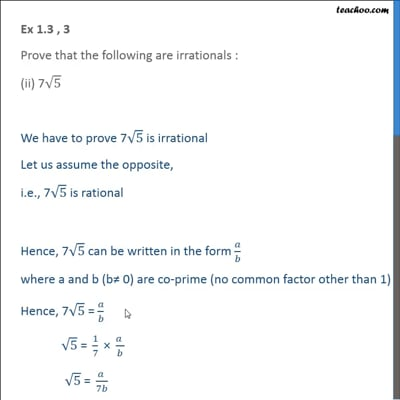Ex 1.3

Chapter 1 Class 10 Real Numbers
Serial order wiseThis video is only available for Teachoo black users

Introducing your new favourite teacher - Teachoo Black, at only ₹83 per month

### Transcript

Ex 1.3 , 3 Prove that the following are irrationals : (ii) 7√5 We have to prove 7√5 is irrational Let us assume the opposite, i.e., 7√5 is rational Hence, 7√5 can be written in the form 𝑎/𝑏 where a and b (b≠ 0) are co-prime (no common factor other than 1) Hence, 7√5 = 𝑎/𝑏 √5 " = " 1/7 " × " (𝑎 )/𝑏 " " √5 " = " (𝑎 )/7𝑏 Here, (𝑎 )/7𝑏 is a rational number But √5 is irrational Since, Rational ≠ Irrational This is a contradiction ∴ Our assumption is incorrect Hence 7√5 is irrational Hence proved